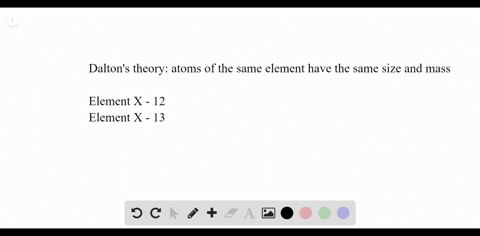### Does the existence of isotopes contradict part of…

00:28University of Miami
Problem 64

Isotopes Are the following elements isotopes of each
other? Explain.
$$_{12}^{24} \mathrm{Mg}_{12}^{25} \mathrm{Mg}_{12}^{26} \mathrm{Mg}$$

Yes.

## Discussion

You must be signed in to discuss.

## Video Transcript

It's a noble work on problem 64 from Chapter four in this problem were asked if some isotopes which are given or isotopes, uh, each other. So first we can write down the isotopes, which we see. So we have 24 12 magnesium, 25 12 magnesium and 26 12 magnesium. So the question is, are the isotopes of each other and the answer would be yes, because they all are the same element hasn't identified by the fact that they all have the same symbol, and they all have the same atomic number. So since they possess the same atomic number, different mass number just means there's different nutrients present, but same number of protons indicates that they are isotopes.